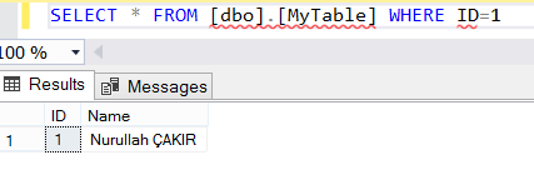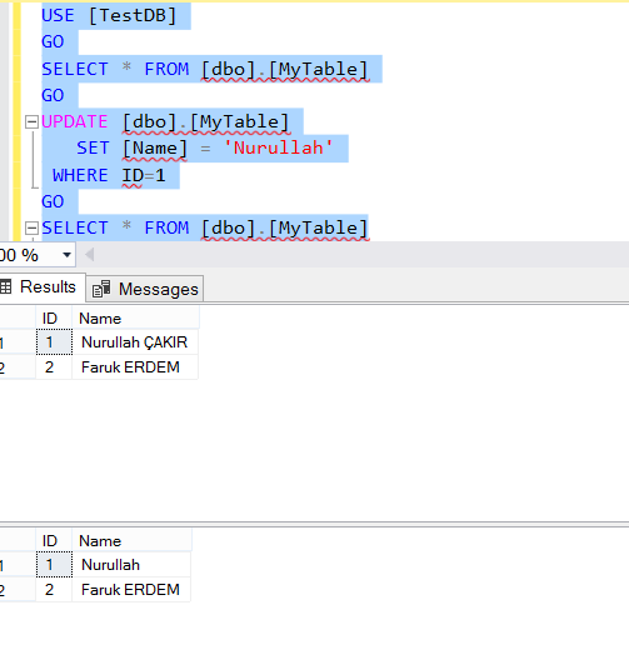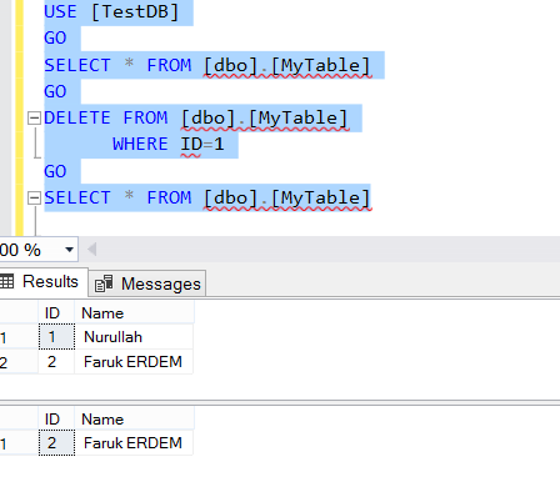Sunday , September 24 2023

# Where Clause in SQL Server and SQL Server Operators(TSQL)

We use WHERE clause to filter when reading, updating, and deleting data from a table. If we run select, Update, or delete statements without using WHERE clause, we will be processing the entire table. Let’s do these operations with an example.

Example:

First, we create a table as below and add a few records to this table.

Using WHERE Clause With SELECT:

We read the records with ID 1 as follows.Using WHERE Clause With UPDATE:

With the following query, we are updating the Name column of the records with ID 1 as “Nurullah”.Using WHERE Clause With DELETE:

We are deleting records with ID 1 from the table with the following query.We can use the following operators in WHERE clause:

Comparison Opertors:

 Where ID=1 If ID is 1 Where ID>1 If ID is greater than 1 Where ID>=1 If ID is 1 and greater than 1 Where ID<1 If ID is less than 1 Where ID<=1 If ID is 1 and less than 1 Where ID<>1 If ID is not 1

“AND” and “OR”:

Logical Operators:

We can use it as follows:

If the Name column has a Nuru expression and the ID is 1

or

If the name column has an ERDEM expression and ID is 2

BETWEEN:

If ID is between 0 and 2

LIKE:

If the name column contains a Nuru expression

IN:

If ID is one of the values of 1,2,3,4

ALL:

If all of the values returned from the subquery meets the condition.

EXISTS:

If a value is returned from the subquery.

NOT:

We use NOT operator with other operators. For example, `"Where Name NOT Like '%Nuru%'"` means that if the name column doenst contains a Nuru expression.

SOME or ANY(They are equivalent):

If any of the values returned from the subquery meets the condition.